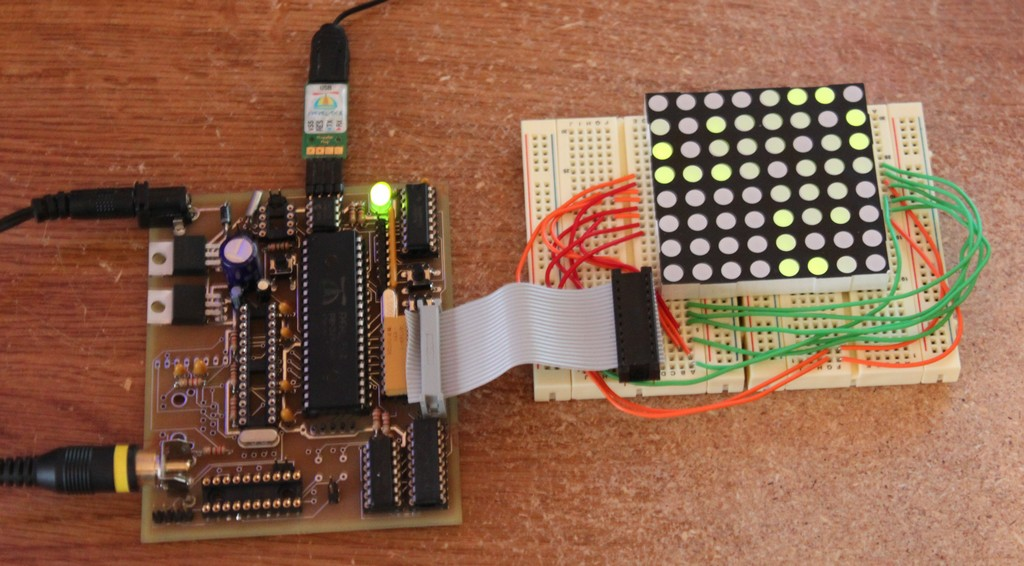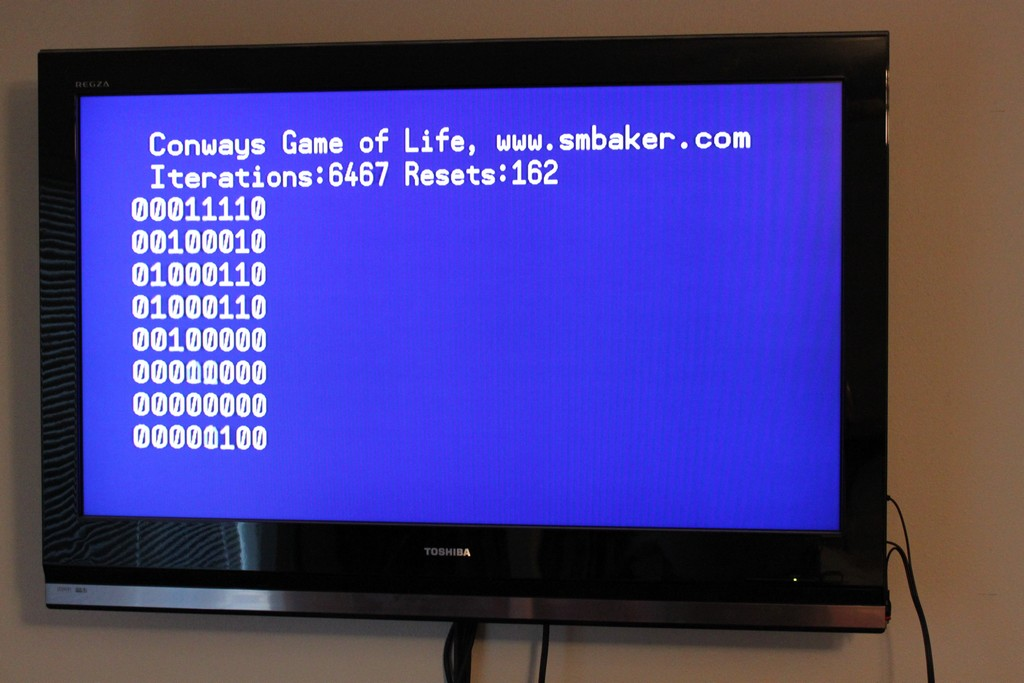# Conway’s game of life on a parallax propeller

I spent an hour or two last night implementing a very simple ‘game of life’ on the parallax propeller embedded microcontroller. My plan is to construct the device using an 8×8 LED array, but for now the proof of context uses the prop’s built-in TV output support to write ones and zeroes to the television. It currently uses the random number generator to setup the game field, and degenerates to a flasher around iteration 250 or so. There’s also some code commented out that will create a slider or a toad.

Here are a couple of screenshots, one showing the game running on my generic clock controller board, connected to a LED matrix, and the other showing what it looks like on the TV:The parallax website has another implementation of Game of Life, using the VGA driver and mouse support. I’ve not tried it, but the main loop is in prop assembly rather than spin, and is probably faster than my unoptimized brute-force implementation.

Below is the source code in spin (the parts for controlling the LED matrix are omitted, as they’re pretty specific to my hardware):

```' Conway's Game of Life
' Implemented for the Parallax Propeller by Dr Scott M Baker
' http://www.smbaker.com/

CON
_clkmode = xtal1 + pll16x
_xinfreq = 5_000_000

OBJ
text  : "TV_Text"

CON
rows = 8
cols = 8
pitch = cols+2
screensize = (rows+2) * (cols*2)

VAR
' The current screen will be held in an array called "cur".
' It is large enough to hold (rows x cols) with an additional 1-cell border
' all the way around. The one-cell border is so that we can easily reflect
' the opposite side to get an infinite playfield.
byte cur[ screensize ]
byte work[ screensize ]
long rnd

PUB main
text.Start(24)
text.out(\$00)

init
tvloop

PUB init | i, x
repeat i from 1 to screensize
byte[@cur + i] := 0

' glider
'byte[@cur + 3*pitch + 5 ] := 1
'byte[@cur + 4*pitch + 3 ] := 1
'byte[@cur + 4*pitch + 5 ] := 1
'byte[@cur + 5*pitch + 4 ] := 1
'byte[@cur + 5*pitch + 5 ] := 1

'byte[@cur + 3*pitch + 3 ] := 1
'byte[@cur + 3*pitch + 4 ] := 1
'byte[@cur + 3*pitch + 5 ] := 1
'byte[@cur + 4*pitch + 2 ] := 1
'byte[@cur + 4*pitch + 3 ] := 1
'byte[@cur + 4*pitch + 4 ] := 1

' just do something random
repeat i from 1 to 25
x := ?rnd
if (x<0)
x:=-x
byte[ @cur + (x // screensize) ] := 1

' copy reflect the edges of the game to the opposite sides (top goes to
' bottom, left to right, etc)
PUB copyborder | i
repeat i from 1 to cols
byte[ @cur+ 0*pitch + i ] :=           byte [ @cur+ rows*pitch + i ]
byte[ @cur+ (rows+1)*pitch + i ] :=    byte [ @cur+ 1*pitch + i ]
repeat i from 1 to rows
byte[ @cur+ i*pitch + 0 ] :=           byte [ @cur+ i*pitch + cols ]
byte[ @cur+ i*pitch + (cols+1) ] :=    byte [ @cur+ i*pitch + 1 ]

byte[ @cur+ 0*pitch + 0 ] :=              byte [ @cur+ rows*pitch + cols ]
byte[ @cur+ 0*pitch + (cols+1) ] :=       byte [ @cur+ rows*pitch + 1 ]
byte[ @cur+ (rows+1)*pitch + 0 ] :=       byte [ @cur+ 1*pitch + cols ]
byte[ @cur+ (rows+1)*pitch + (cols+1)] := byte [ @cur+ 1*pitch + 1 ]

' update the game by applying the game of life rules.
PUB update | r, c, live, liven, i, livec, changes, ptr
copyborder

livec := 0
changes := 0

repeat r from 1 to rows
repeat c from 1 to cols
live := byte [ @cur+ r*pitch + c ]

' count the number of live neighbors, starting with the top left corner
ptr := @cur + (r-1) * pitch + (c-1)
liven := byte[ptr] + byte[ptr+1] + byte[ptr+2] + byte[ptr+pitch] + byte[ptr+pitch+2]
liven := liven + byte[ptr+pitch*2] + byte[ptr+pitch*2+1] + byte[ptr+pitch*2+2]

if (live==1) and (liven>1) and (liven<4)
' if a live cell has 2-3 live neighbors, it stays live
byte[ @work+ r*pitch + c ] := 1
livec := livec + 1
elseif (live==0) and (liven == 3)
' if a dead cell has exactly three neighbors, it becomes live
byte[ @work+ r*pitch + c ] := 1
livec := livec + 1
changes := changes + 1
elseif (live==1)
' otherwise if a live cell has less than 2 it dies of starvation
' or greater than 4, it dies of overcrowding
byte[ @work+ r*pitch + c ] := 0
changes := changes + 1

' copy the work array back into the screen array
repeat i from 0 to (screensize-1)
byte[@cur + i] := byte[@work + i]

' if the whole game is dead, or if nothing is changing, restart
if (livec == 0) or (changes == 0)
init

PUB tvloop | r, c, count
count := 0
repeat
update
text.str(string(\$A, 1, \$B, 1, "Conways Game of Life, www.smbaker.com"))
text.str(string(\$A, 1, \$B, 2, "Iterations:"))
text.dec(count)
text.out(13)
repeat r from 1 to rows
repeat c from 1 to cols
text.dec( byte[ @cur + r*pitch + c ] )
text.out(13)

count:=count+1

waitcnt( clkfreq/20 + cnt )```